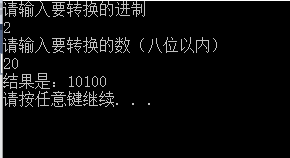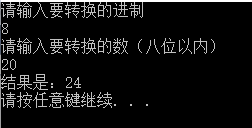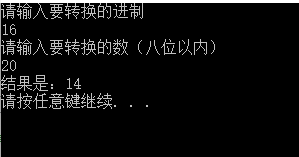• 十进制数转16进制数字源代码，VB程序，演示测试用
• 后来领导一句话，让我明白了，“十进制数16进制数是一样的”，确实，貌似，刚来时，用C++对公司原有的项目进行维护时，增加过一个标定功能，给发送数组赋的就是10进制的说。 Data_volt = (byte)(0x80 + (...
最近在做一个项目，其中发送部分需要对数据进行移位和&操作，在网上找了许久的转换方式，结果并没有。后来领导一句话，让我明白了，“十进制数和16进制数是一样的”，确实，貌似，刚来时，用C++对公司原有的项目进行维护时，增加过一个标定功能，给发送数组赋的就是10进制的说。

Data_volt = (byte)(0x80 + ((Convert.ToByte("0x" + v_data.Substring(0, 2), 16)) & 0x7f));

以后还是要多总结，不然一些傻乎乎的事儿，做起来还风风火火的。

附上查找资料过程中有用的一些资料地址：

https://www.cnblogs.com/ThreeS/articles/9377547.html

https://www.cnblogs.com/screes/p/5633383.html

还有一个关于补零的：https://blog.csdn.net/qq_22889875/article/details/79320678

参考其我如此使用：

 //将16进制字符串低位字节补零
//string str= Convert.ToString(a, 16);
//str为整数a对应的十六进制字符串
public string HexstrAddZero(string str)
{
string strByeZero = "";
if(str.Length == 4)
{
strByeZero = str;
}
else if(str.Length == 3)
{
strByeZero = "0"+str;
}
else if (str.Length == 2)
{
strByeZero = "00" + str;
}
else if (str.Length == 1)
{
strByeZero = "000" + str;
}
else if(str.Length == 0)
{
strByeZero = "0000";
}
return strByeZero;
}

扩展为两个字节的16进制字符串补零
展开全文• 进制转换
环境：win10，vs2013
此文用来把一个十进制整数转换为一个输入的进制（最大为十六进制）所对应的的数，即就是输入十进制数可以转换为任意进制

#define _CRT_SECURE_NO_WARNINGS
#include <stdio.h>
#include <stdlib.h>
void translate(long num, int transformRight)
{
int number = { '0', '1', '2', '3', '4', '5', '6', '8', '9', 'a', 'b', 'c', 'd', 'e', 'f' };
int numIndex;
int i = 0;
while (num>0 && i<8)
{
numIndex[i] = num%transformRight;//存放余数
num /= transformRight;//变化"num"的值
i++;

}
for (i -= 1; i >= 0; i--)//逆序输出余数（即就是运算后的结果）
{
switch (numIndex[i])
{
case 0:
case 1:
case 2:
case 3:
case 4:
case 5:
case 6:
case 7:
case 8:
case 9:printf("%d", numIndex[i]); break;
case 10:printf("a"); break;
case 11:printf("b"); break;
case 12:printf("c"); break;
case 13:printf("d"); break;
case 14:printf("e"); break;
case 15:printf("f"); break;
}

}
printf("\n");
}
int main()
{
int num, transformRight;
printf("请输入要转换的进制\n");
scanf("%d", &transformRight);
printf("请输入要转换的数（八位以内）\n");
scanf("%d", &num);
printf("结果是：");
translate(num, transformRight);
system("pause");
return 0;
}展开全文进制转换
• m进制数转十进制数（乘权取余法）#include<iostream> #include<cmath> using namespace std; int main(){ int n,m; int ans=0; cin>>n>>m; for(int i=6;i>=0;i--){ int a=n...
m进制数转十进制数（乘权取余法）
#include<iostream>
#include<cmath>
using namespace std;
int main(){
int n,m;
int ans=0;
cin>>n>>m;
for(int i=6;i>=0;i--){
int a=n/pow(10,i);   //1式
int b=n/pow(10,i+1);  //2式
int c=pow(m,i);      // 3式
ans+=(a-b*10)*c;         //4式
}
cout<<ans<<endl;
return 0;
}

1~4式主要是为了单步调试方便，可以写成一步。
但这只对于10进制以内的数转为十进制数有作用。比如16进制中单位存在A~F用int型则不能准确表示了。
为适应更高数制，修正后的代码如下（当然为了题目要求这里最高数制可以转换到16进制数）：
#include<iostream>
#include<cmath>
#include<string>
using namespace std;
int main(){
string n;
int m;
int ans=0;
cin>>n>>m;
int q=n.size();
for(int i=0;i<q;i++){
if(n[i]<=57&&n[i]>=48)
ans+=(n[i]-48)*pow(m,q-i-1);
if(n[i]<=70&&n[i]>=65)
ans+=(n[i]-55)*pow(m,q-i-1);
if(n[i]<=102&&n[i]>=97)
ans+=(n[i]-87)*pow(m,q-i-1);
}
cout<<ans<<endl;
return 0;
}

十进制数转m进制数（反向取余法）
#include<iostream>
#include<cmath>
#include<string>
using namespace std;
int main(){
int n,m;
cin>>n>>m;
char num;
int res=n,i=0,cnt,p;
while(res!=0){
res=n/m;
p=n%m;
if(p==10)num[i++]='A';
else if(p==11)num[i++]='B';
else if(p==12)num[i++]='C';
else if(p==13)num[i++]='D';
else if(p==14)num[i++]='E';
else if(p==15)num[i++]='F';
else num[i++]=p+48;
n=res;
}
cnt=i;
for(i=cnt-1;i>=0;i--)
cout<<num[i];
cout<<endl;
return 0;
}



展开全文• 可以完成16进制数转换成十进制16进制转换，使用vb语言，方便好用
• 进制转换：十进制数转十六进制数 问题描述 十六进制数是在程序设计时经常要使用到的一种整数的表示方式。它有0,1,2,3,4,5,6,7,8,9,A,B,C,D,E,F共16个符号，分别表示十进制数的0至15。十六进制的计数方法是满16进1，...
进制转换：十进制数转十六进制数
问题描述

十六进制数是在程序设计时经常要使用到的一种整数的表示方式。它有0,1,2,3,4,5,6,7,8,9,A,B,C,D,E,F共16个符号，分别表示十进制数的0至15。十六进制的计数方法是满16进1，所以十进制数16在十六进制中是10，而十进制的17在十六进制中是11，以此类推，十进制的30在十六进制中是1E。
给出一个非负整数，将它表示成十六进制的形式。

代码描述（一）
#include <stdio.h>
int main()
{
int n,i,j=0;
char a={'0','1','2','3','4','5','6','7','8','9','A','B','C','D','E','F'};//*创建一个十进制与十六进制对应的数组，并对其赋值*//
char b;//*存放已转换好的十六进制的数组长度未知，自定义的长度32*//
scanf("%d",&n);
while(n>=16)
{
i=n%16;
b[j]=a[i];
j++;//*新的数组的下标移位*/
n=n/16;
}//*该while循环体的作用是把n大于16的数转换十六进制数再依次输入新的数组中*//
if(n<16)
b[j]=a[n];
//*注意：此时数组b[]内的十六进制数是倒过来存储的*//
while(j>=0)
{
if(j>0)//*判断是不是最后一个，不是的话输出，是的话直接换行*//
printf("%c",b[j]);
else
printf("%c\n",b[j]);
j--;
}
return 0;
}

代码描述（二）
#include <stdio.h>
int main()
{
int n, i, j = 0;
char a = { '0','1','2','3','4','5','6','7','8','9','A','B','C','D','E','F' };
char b;
scanf("%d", &n);
if (n ==0)
printf("0\n");
while (n)
{
i = n % 16;
b[j] = a[i];
++j;
n = n / 16;
}
for (i = j; i > 0; i--)
printf("%c", b[i-1]);
printf("\n");
return 0;
}



展开全文c语言
• 类似十进制数转二进制数，除以16，得到的余数倒着写出来即可。 例如： 673÷16=42……1 42÷16=2……10（A） 2÷16=0……2 所以，673(10)=2A1(16) 余数中0—9仍然用0—9表示，而10—15用A—F表示java
• 十六进制转换成十进制Data SEGMENT String DB 'input 4 hex num:',0ah,0dh,'\$' Num DB 10 DUP(?) Data ENDS Code SEGMENT ASSUME CS:code,DS:data Start: MOV AX,daTA MOV DS,AX LEA DX,string MOV AH ,9 INT 21h ...
• 十进制数转换为十六进制数Conversion of decimal number system into hexadecimal number system can be done by successively dividing an integral part by 16 till the quotient is 0 and then reading the ...clang printf java vba bluetooth
• 已知：十进制数123被转换...//10进制数转16进制 System.out.println("请输入一个整数："); Scanner in =new Scanner(System.in); int number =in.nextInt(); String hex =""; int shang =number; int yu; whilejava
• kotlin 16进制Given a number in decimal number system format, we have to convert it into octal number system format. 给定一个十进制数字格式的数字，我们必须将其转换为八进制数字格式。 Example: 例： ...
• 将十六进制数转换成十进制数。...3、将转换的十进制数乘以16的n次方； 4、相加。 #include #include #include int fun (char ch) { int a; switch (ch) { case 'A': return 10; //A~F单独转换
• 展开全部一、转换的32313133353236313431303231363533e59b9ee7ad9431333365653163思路分析：4个2进制位为一个16进制数，2进制1111为16进制F，2进制中千位的1=8，百位的1=4，位的1=2，个位的1=1，将各个位的数作...
• 这是一个将十进制数字转化成十六进制的数字的讲解，当然稍微改一下也适用于其他进制数之间的转化。 十六进制数字包含十进制数字0、1、2、3、4、5、6、7、8、9以及表示十进制数字10的A，表示十进制数字11的B,表示12...
• 十进制数除以其他进制取余反序排列 #include #define BASE 16 //要转换的进制类型 int trans (int n) { if (n == 0) return; trans (n/BASE); //递归取整 if (n%BASE > 9) //取余打印 printf ("%...
• 001将十进制数转换为二进制数1.代码如下：2.个人见解：3.符号解释： 1.代码如下： #include<stdio.h> int main(void) { int i, j, m, n; int arr = { 0 }; printf("请输入一个十进制数：\n"); scanf(...
• 后来又接触了8进制，16进制，慢慢明白，这些都只是数值的一种表达形式，10进制更容易理解和使用，所以我们平时使用10进制。在编程中，进制转换是很常见的操作，那么今天，就和大家一起来实现10进制到36进制的转换。...
• kotlin 16进制Given a number in octal number system format, we have to convert it into decimal number system format. 给定八进制系统格式的数字，我们必须将其转换为十进制系统格式。 Example: 例： ...tensorflow java docker 深度学习
• 输入一个十进制数，将其转换为十六进制数并输出。 #将十进制转换为十六进制 def decimalToHex(decValue): hex="" while decValue !=0: hexValue=decValue%16 #求余数 hex=toHexChar(hexValue)+hex decValue=...
• 用最基础的方法实现两位十进制转16进制输出，原创资源，如有疑问，欢迎交流！
• PAGE PAGE #/ 4 实验课题一将一个十进制数转换成另外一个 P 进制数字符串(可以是二 进制到十六进制) 转换函数的原 型为 void Convert (int n,char str[], unsigned P; n输入待转换的数 str :输出转换好的P进制字符串...
• 例：我们将728这个十进制数转换为4位十进制表示。通常用于数码管的显示。 首先我们定义一个比较寄存器comp，因为转换的值为728，所以定义一个16位的寄存器2^16=1024*64（偏大）。 在定义4个转换寄存器，4位足够。 ...fpga
• 思路：先将输入的十进制数除以16得到商和余数，再将得到的商继续除以16得到商和余数，以此类推直到商为0为止，然后将所得余数顺序存入一个数组，再将这个数组倒序通过switch语句依次成十六进制数存入一个字符串...C语言 基础训练
• 实验课题一 将一个十进制数转换成另外一个P进制数字符串可以是二进制到十六进制转换函数的原 型为 void Convert (int n, char str[], unsigned P; n输入待转换的数 str输出转换好的P进制字符串 P输入要转换的进制...
• 该楼层疑似违规已被系统折叠隐藏此楼查看此楼'在编辑框"ten"(需要设置输入方式"输入数字")中输入10进制数字,编辑框"sixteen"中输出16进制数字过程 十转十六()变量 寄存ten 为 整数型sixteen.内容 =""如果 ten.内容 &......